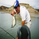1054 views
1054
I am republishing to use a clean chart. Previous one had too much of a mess. Idea for TradingView: Please allow us to change out the charts after publishing.

I like to know when volume spikes (only when it spikes). I am not interested in seeing the rest of the volume bars. I created this indicator to show me when it explodes (i.e. the name "Volume Bomb" , plus it sounds cool).
This indicator only shows you when volume exceeds the EMA of volume by whatever multiplier you set.
Default settings are the current volume with 10 EMA . Yellow arrowup will appear when volume is at 1.5x the 10 EMA .
```//Created by Robert N. 033015
// This indicator only shows you when volume exceeds the EMA of volume by whatever multiplier you set
// Default settings are the current volume with 10 EMA.  Yellow arrowup will appear when volume is at 1.5x the 10 EMA

study(title="Volume Bomb", precision=0, overlay = false)
EMovingAvg = ema(volume,input(10))
Multiplier=(volume >= (EMovingAvg * input(1.5)))
plotshape(Multiplier, title="Vol", text='Vol', style=shape.arrowup, location=location.bottom, color=yellow)

```Dear Sir, below is volume indicator code in version 4, I am trying to club with other indicator which is in version 3, so I am getting error. Can you pls suggest what changes I need to make in below code to work:

BarsVisible = input(title="Bars Visible", type=input.bool, defval=true)

SmoothPeriod = input(30, title="Smooth Period")
SmoothMAType = input(title="Smooth MA Type", defval="HMA", options=)
SmoothVisible = input(title="Smooth MA Visible", type=input.bool, defval=true)

AbsoluteEffectiveness = input(title="Absolute Effectiveness", type=input.bool, defval=false)

VolumePower = if(AbsoluteEffectiveness)
abs((((close / open) - 1) * 100)/volume)
else
(((close / open) - 1) * 100)/volume

ma(MAType, MASource, MAPeriod) =>
if MAType == "SMA"
sma(MASource, MAPeriod)
else
if MAType == "EMA"
ema(MASource, MAPeriod)
else
if MAType == "WMA"
wma(MASource, MAPeriod)
else
if MAType == "RMA"
rma(MASource, MAPeriod)
else
if MAType == "HMA"
wma(2*wma(MASource, MAPeriod/2)-wma(MASource, MAPeriod), round(sqrt(MAPeriod)))
else
if MAType == "DEMA"
e = ema(MASource, MAPeriod)
2 * e - ema(e, MAPeriod)
else
if MAType == "TEMA"
e = ema(MASource, MAPeriod)
3 * (e - ema(e, MAPeriod)) + ema(ema(e, MAPeriod), MAPeriod)
else
if MAType == "VWMA"
vwma(MASource, MAPeriod)

MA = ma(SmoothMAType, VolumePower, SmoothPeriod)

plot(BarsVisible ? VolumePower : na, color=(VolumePower>=0 ? color.green : color.red), style=plot.style_columns, transp=50 )
plot(SmoothVisible ? MA : na, color=color.black, style=plot.style_line)

-------------------------------------------------------------------------------------------------------------------------------------------------------------------

Regards,
Vishwanath